# High School Math : Finding Limits as X Approaches Infinity

## Example Questions

### Example Question #1 : Finding Limits As X Approaches Infinity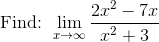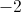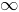Explanation: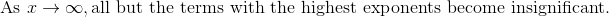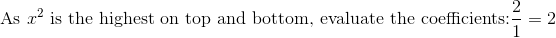### Example Question #5 : Limits

Calculate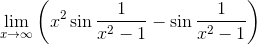.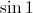The limit does not exist.Explanation:

This can be rewritten as follows: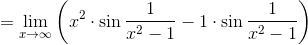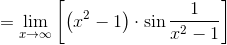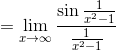We can substitute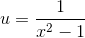, noting that as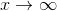,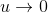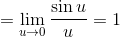, which is the correct choice.

### Example Question #2 : Find The Limit Of A Function

The speed of a car traveling on the highway is given by the following function of time: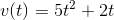What can you say about the car's speed after a long time (that is, asapproaches infinity)?

The speed of the car depends on the starting speed.

The speed of the car approaches zero.

The speed of the car approaches infinity.

The speed of the car approaches a constant number.

Nothing can be concluded from the given function.

The function given is a polynomial with a term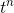, such thatis greater than 1.
Whenever this is the case, we can say that the whole function diverges (approaches infinity) in the limit asapproaches infinity.
This tells us that the given function is not a very realistic description of a car's speed for large!